# 5.1   Formal Languages

In this section, we introduce formal languages, regular expressions, deterministic finite state automata, and nondeterministic finite state automata.

## Basic definitions.

We begin with some important definitions.
• A symbol is our basic building block, typically a character or a digit.
• An alphabet is a finite set of symbols.
• A string is a finite sequence of alphabet symbols.
• A formal language is a set of strings (possibly infinite), all over the same alphabet.
Now, we consider some examples.
• Binary strings. We begin with examples of formal languages over the binary alphabet. The simplest way to specify a formal language is to enumerate its strings. One complication is that languages can be large or infinite sets, so we often use informal descriptions.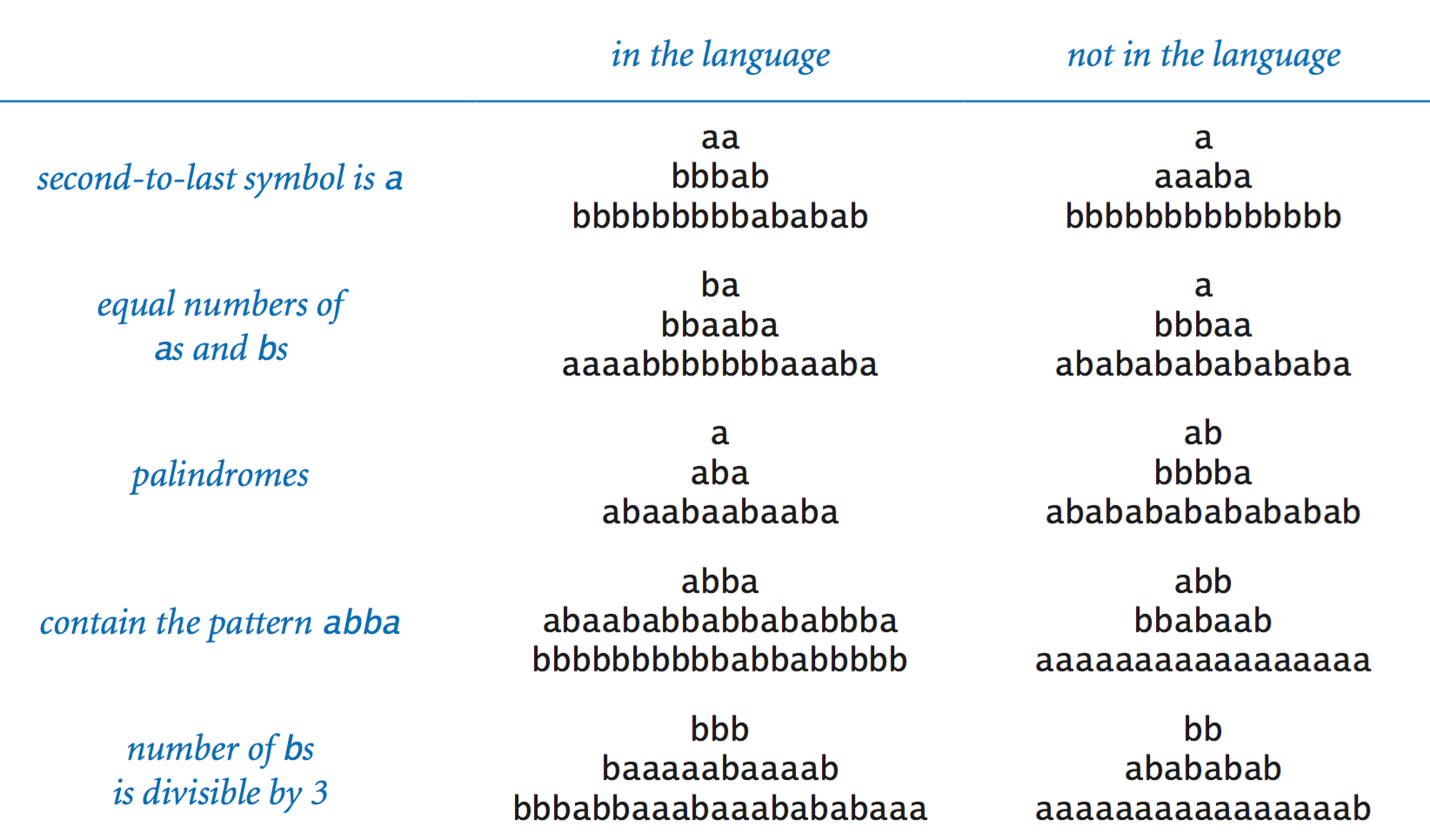• Other alphabets. We use whichever alphabet is suitable to the task at hand when working with formal languages: the standard Roman alphabet for processing text, decimal digits for processing numbers, the alphabet { A, T, C, G } for processing genetic data, and so forth.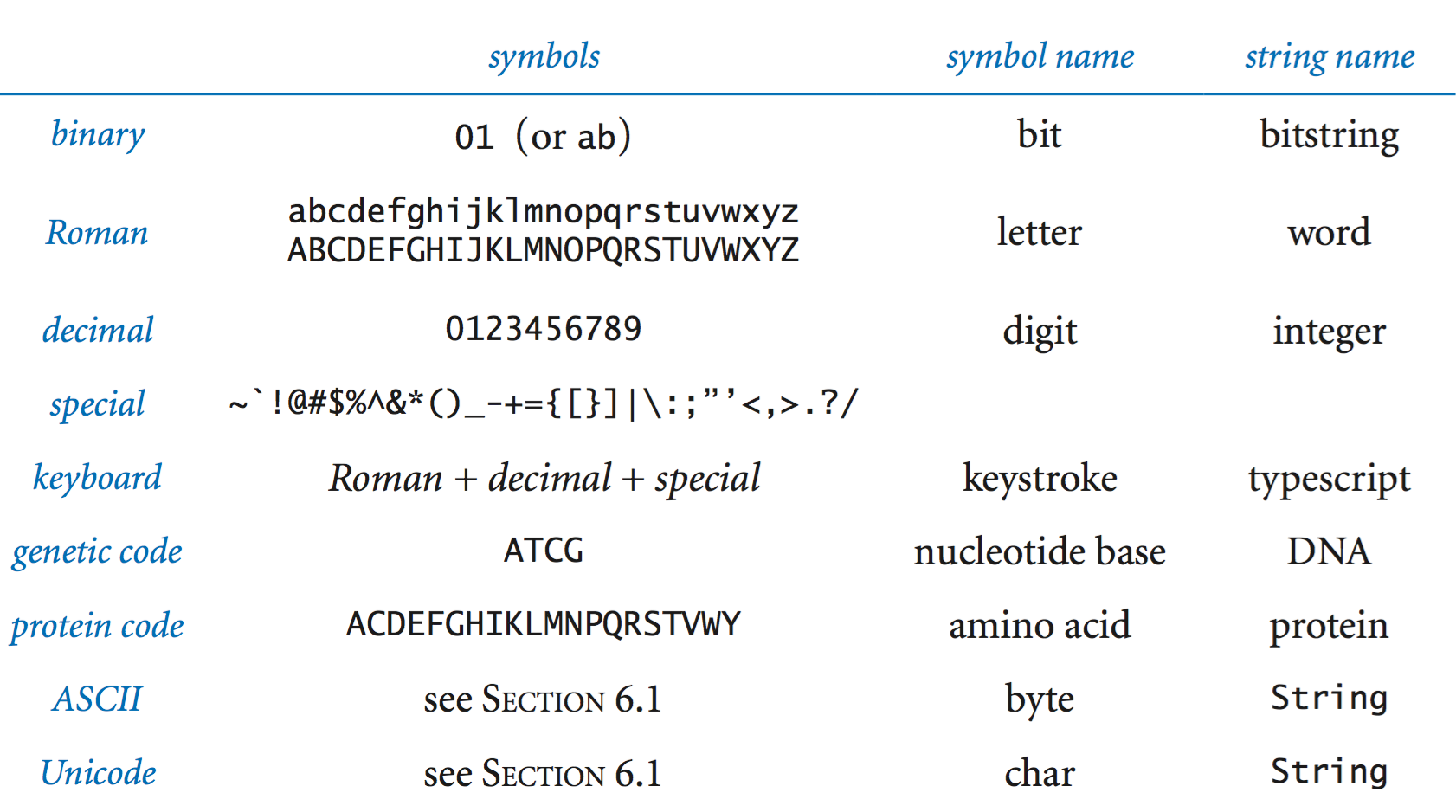Here are some example languages over different alphabets: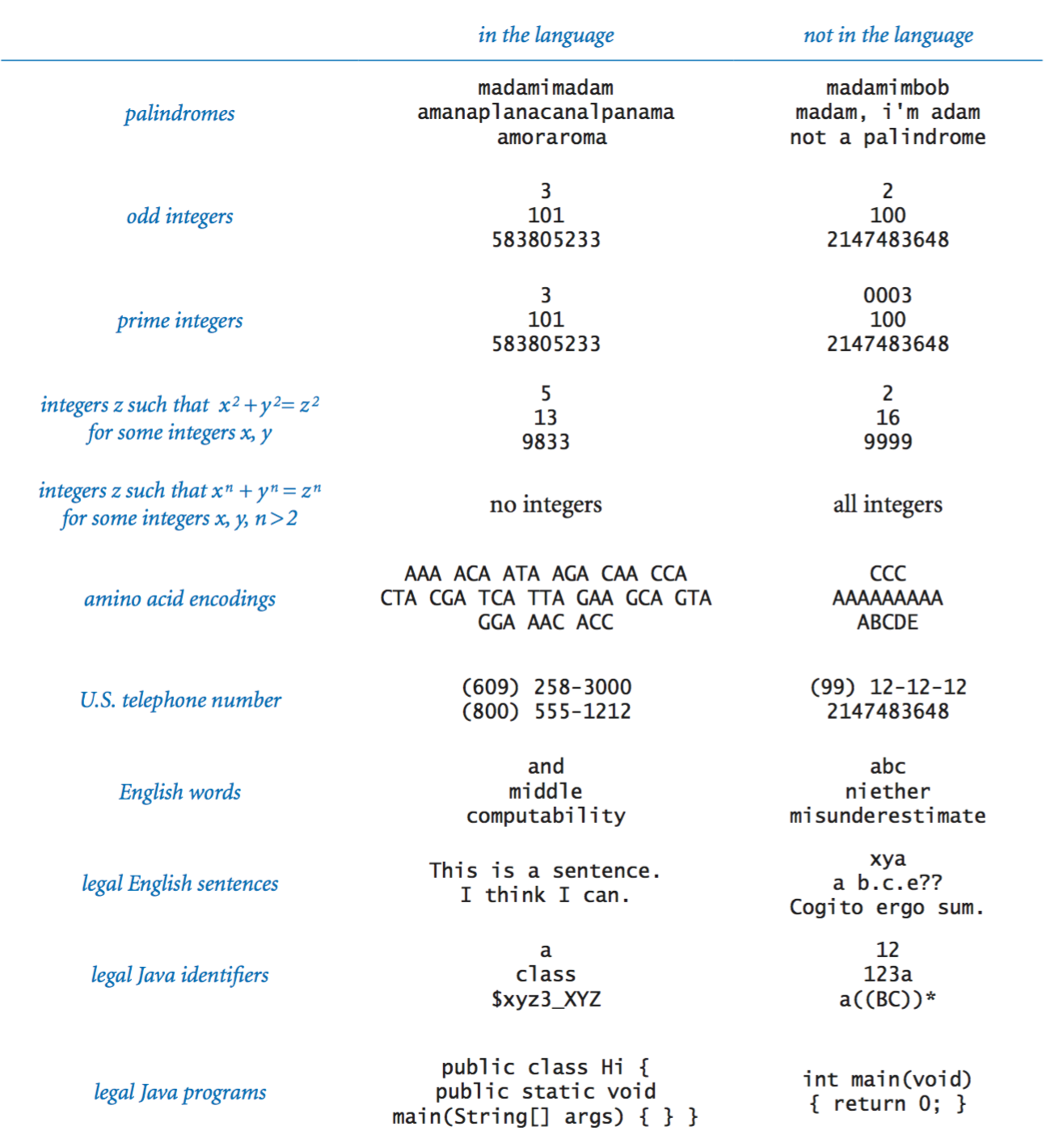• Specification problem. How do we completely and precisely define formal languages? This task is known as the specification problem for formal languages. Our informal English-language descriptions do the job in some cases but are rather inadequate in others.
• Recognition problem. Given a language L and a string x, the recognition problem is to answer the following question: Is x in L?

## Regular languages.

We now consider an important class of formal languages known as the regular languages, for which we can solve the specification and recognition problems.
• Basic operations. We use the union, concatenation, and closure operations on sets, along with parentheses, to specify a regular language.
• The union R | S of two formal languages R and S is the set of strings that are in either R or S (or both). For example,
 { a, ba } | { ab, ba, b } = { a, ab, ba, b } 
We do not include duplicates in the union.
• The concatenation RS of two formal languages R and S is the set of all strings that can be created by appending a string from R to a string from S. For example,
 { a, ab } { a, ba, bab } = { aa, aba, abab, abba, abbab } 
Again, we do not include duplicates in the result.
• The closure R* of a formal language R is the concatenation of zero or more strings from R.
 R* = ε | R | RR | RRR | RRRR | RRRRR | RRRRRR ... 
Note that each time we take a string from R, we are free to use any string in the set. Here ε refers to the empty string—the string consisting of 0 characters.
• We use parentheses or rely on a defined operator precedence order to specify in which order should the operators be applied. For REs, closure is performed before concatenation, and concatenation is performed before union.
• Regular expressions.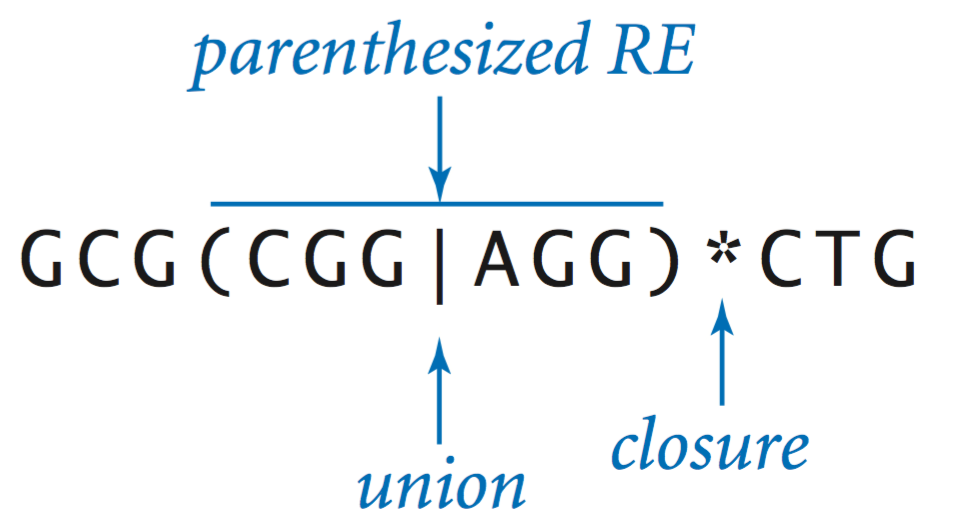A regular expression (RE) is a string of symbols that specifies a formal language. Every regular expression is either an alphabet symbol, specifying the singleton set containing that symbol, or composed from the following operations (where R and S are REs):
• Union: R | S, specifying the union of the sets R and S,
• Concatenation: RS, specifying the concatenation of the sets R and S,
• Closure: R*, specifying the closure of the set R,
• Parentheses: (R), specifying the same set as R.
• Regular languages. A formal language is regular if and only if it can be specified by an RE. Here are some examples: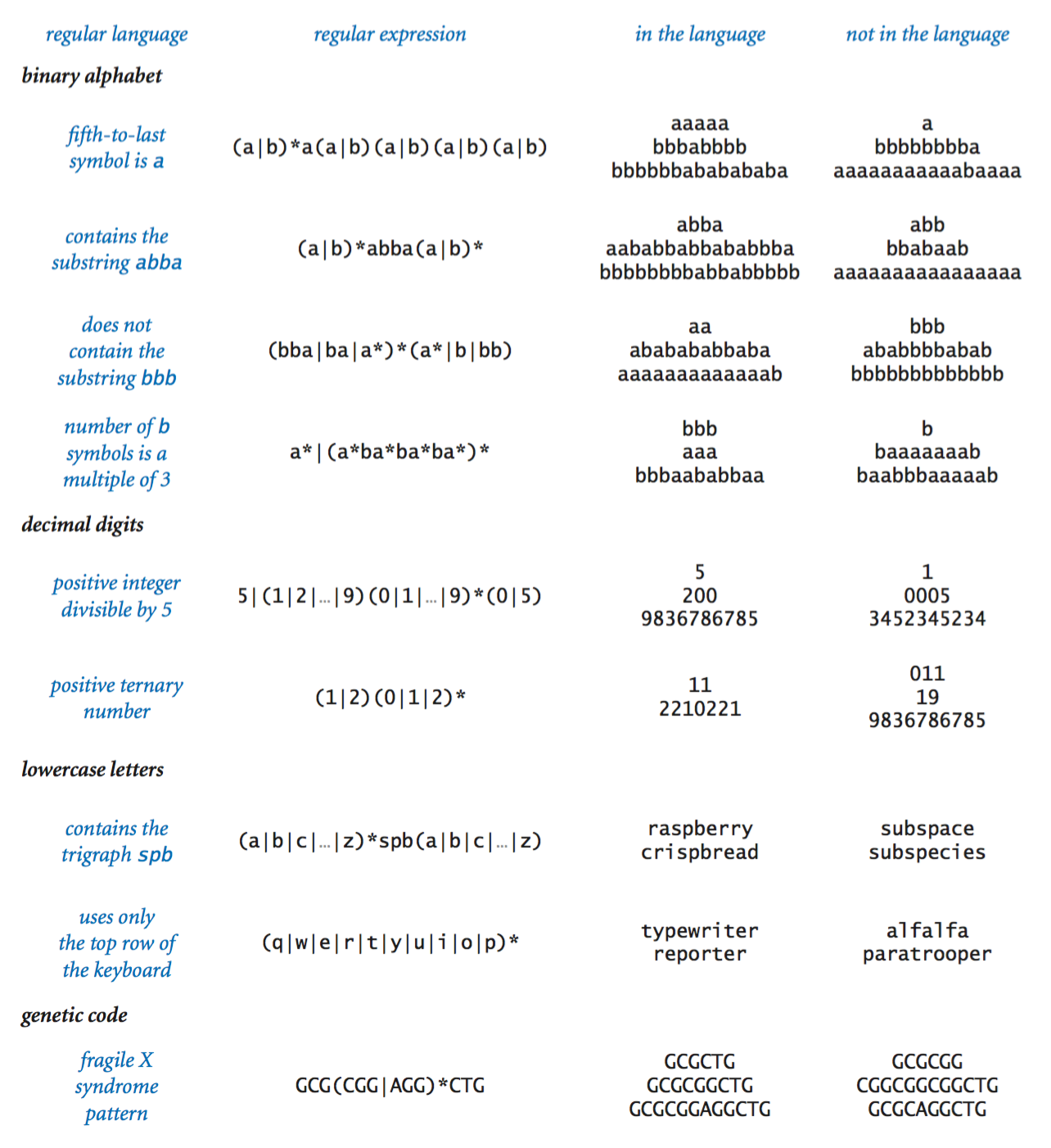## Generalized REs.

Our definition of REs is a minimal one that includes the four basic operations that characterize regular languages (concatenation, union, closure, and parentheses). In practice, it is useful to make various additions to this set.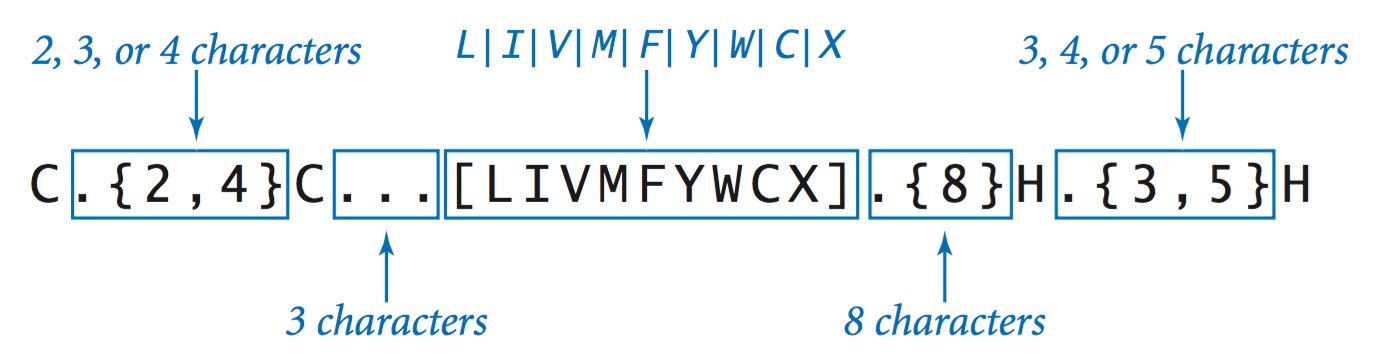• Expanding the alphabet. We need an escape mechanism to allow us to use the metasymbols |, *, (, and ) both to specify REs and as symbols in the language alphabet. Specifically, to use a metasymbol as a symbol in the alphabet, we precede it with the backslash character (/).
• Shorthand notations. Generalized REs support a number of shorthand notations, such as the following:
• The wildcard symbol . matches any alphabet symbol.
• The metasymbol ^ matches the beginning of a line and $matches the end of a line. • A list or range of symbols enclosed in square brackets [] matches any symbol in the list or range. • If the first character within the square braces is the ^ character, the specification refers to the Unicode characters not in the list or range. • Several escape sequences consisting of a backslash followed by an alphabet symbol match a defined set of symbols. For example, \s matches any whitespace symbol. • Extensions to the closure operation. Accordingly, Java REs have the following option for specifying restrictions on the number of repetitions of the closure operation: • One or more: + • Zero or one: ? • Exactly n: {n} • Between m and n: {m, n} The following table illustrates several of these shorthand notations: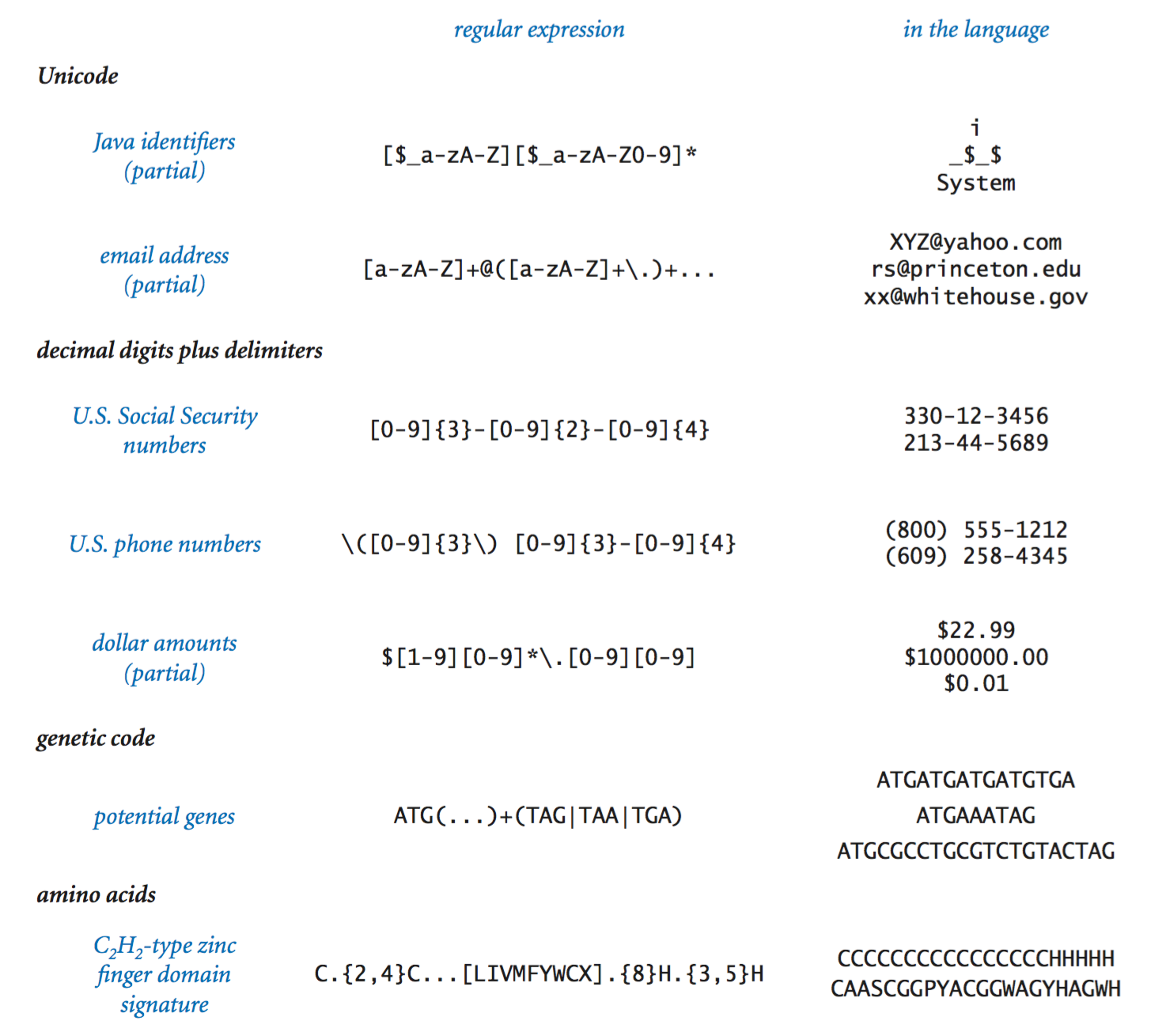Java includes many more shorthands and extensions that we will not explore. ## Regular expressions in Java. The matches() method in Java’s String library solves the recognition problem for generalized regular expressions. If s is any Java String and re is any regular expression, then s.matches(re) is true if s is in the language specified by re, and false otherwise. • Validity checking. Validate.java takes an RE as a command-line argument and, for each string on standard input, prints Yes if it is in the language specified by the RE, and No if it is not. • Searching. Grep.java takes an RE as a command-line argument and prints to standard output all the lines in standard input having a substring in the language described by the RE. ## Deterministic finite-state automata. A DFA is an abstract machine that consists of • A finite number of states, each of which is designated as either an accept state or a reject state. • A set of transitions that specify how the machine changes state. Each state has one transition for each symbol in the alphabet. • A tape reader initially positioned at the first symbol of an input string and capable only of reading a symbol and moving to the next symbol. We represent each DFA as a directed graph where accept states are vertices labeled Yes, reject states are vertices labeled No, and each transition is a directed edge that is labeled with a symbol from the alphabet.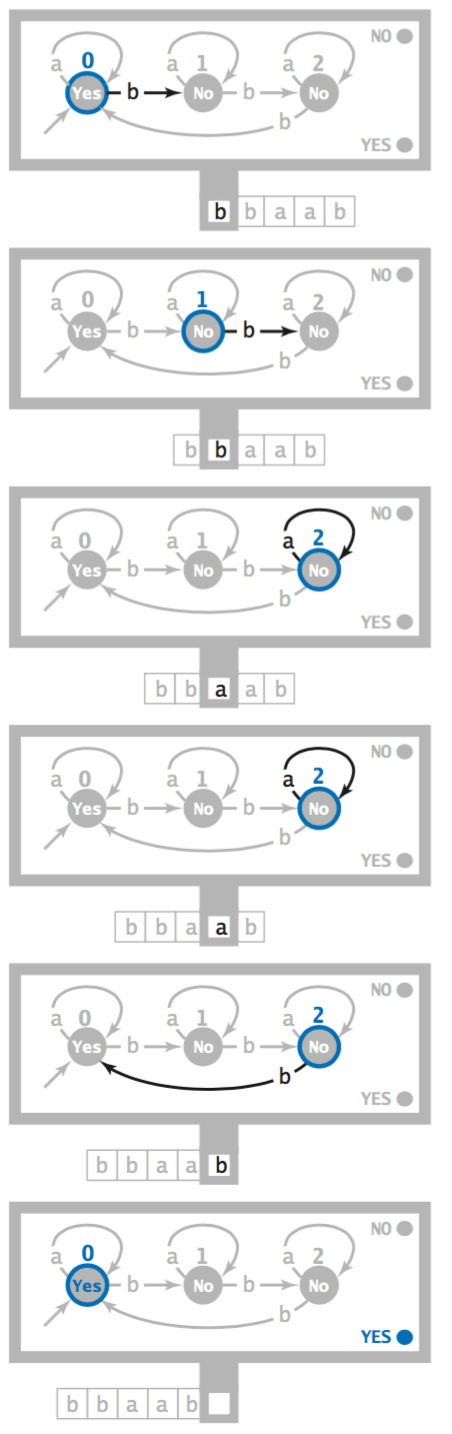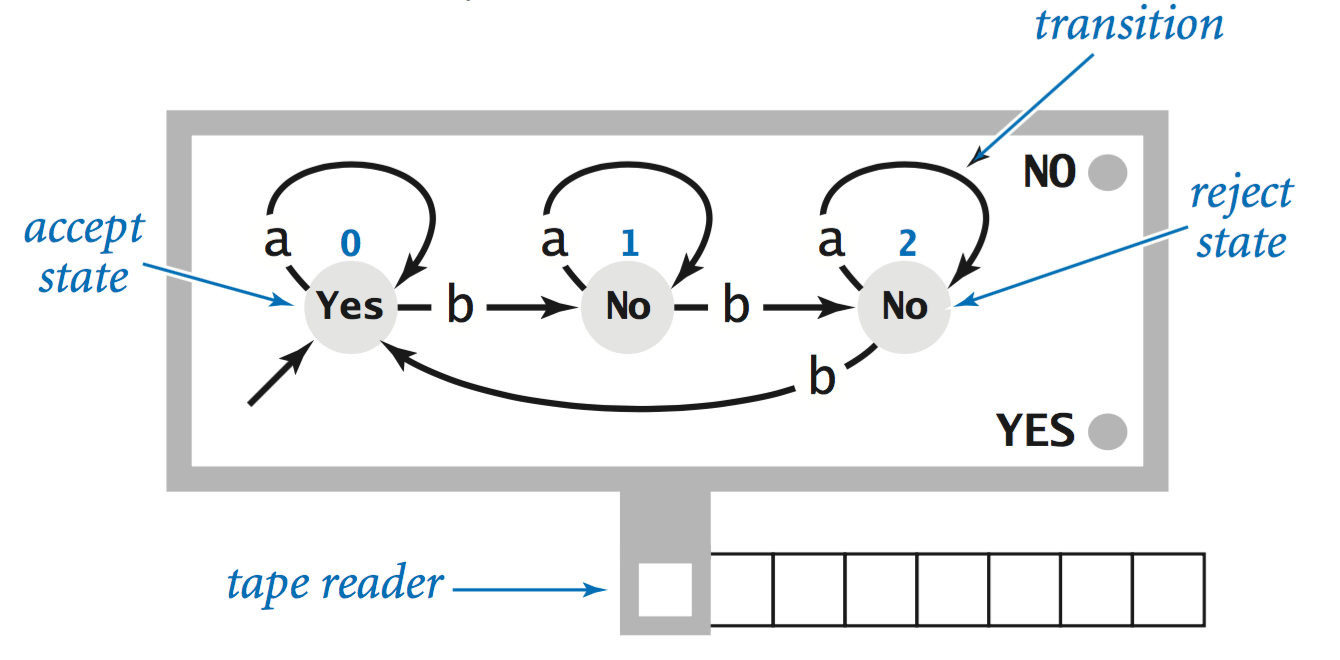• Operation. All DFAs start at state 0 with an input string on the tape and the tape head on the leftmost symbol in the input string. The machine operates by reading a symbol, moving the tape head right one position, and then changing state as specified by the transition labeled with the input symbol just read. When the input is exhausted, the DFA halts. • Characterizing the language. Each DFA recognizes a formal language—the set of all strings it accepts. For example, the above DFA recognizes all binary strings for which the number of bs is a multiple of 3. • Java implementation. DFA.java takes a DFA specification (from a file named on the command line) and a sequence of strings from standard input and prints the result of running the DFA on the given input strings. ## Nondeterministic finite-state automata. The behavior of a DFA is deterministic: for each input symbol and each state, there is exactly one possible state transition. A nondeterministic finite automaton (NFA) is the same as a DFA, but with restrictions on the transitions leaving each state removed, so that • Multiple transitions labeled with the same symbol are allowed. • Unlabeled state transitions (null transitions) are allowed. Following a null transition does not consume an input symbol. • Not all symbols need be included among the transitions leaving each state. An NFA accepts a string if there is any sequence of transitions that can take the machine from the start state to an accept state.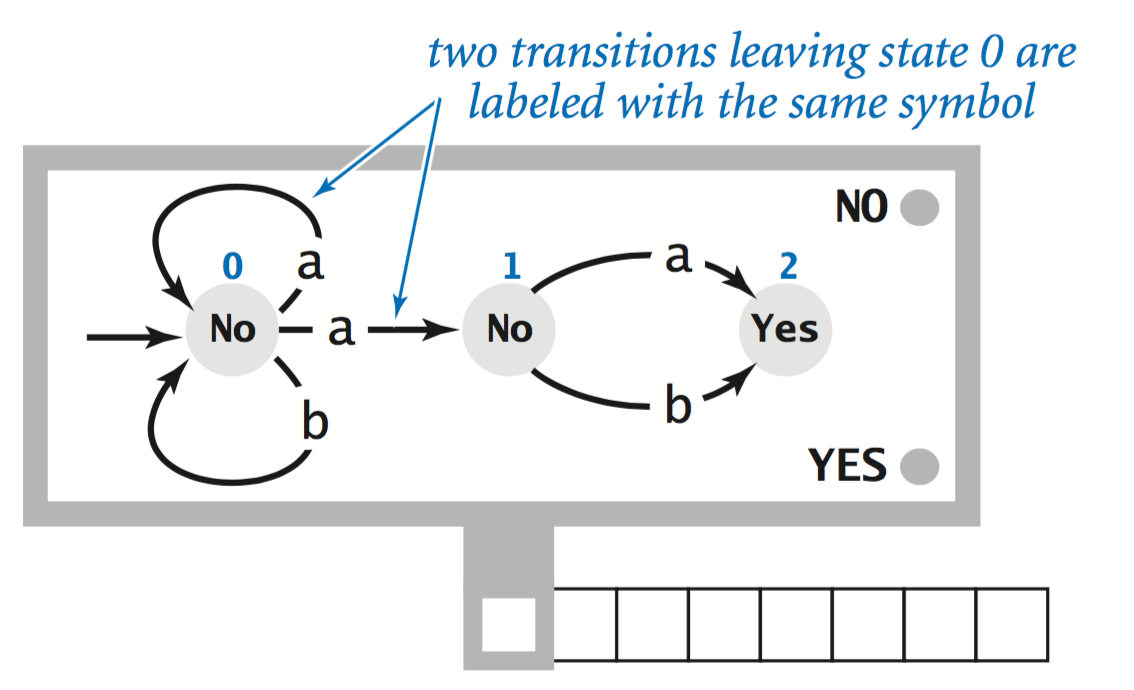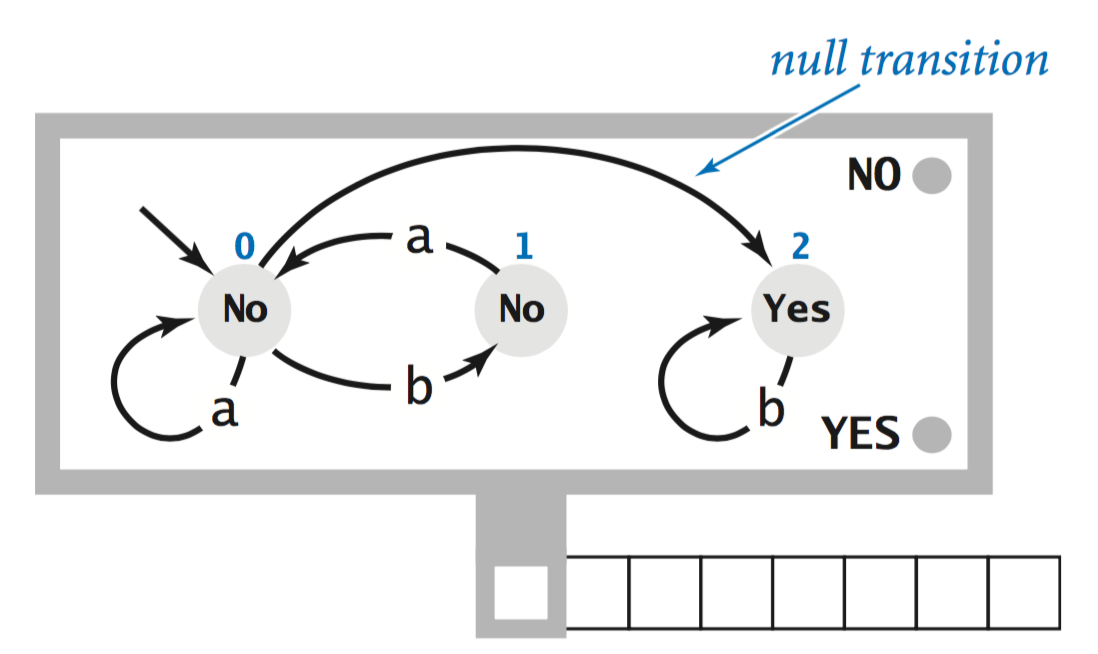• In the NFA at left, when in state 0 and reading an a, it can choose to stay in state 0 or make the transition to state 1. It recognizes binary strings whose second-to-last symbol is a. • In the NFA at right, when it state 0, it can follow the null transition to state 1, without consuming an input symbol. It recognizes binary strings that do not contain the substring bba. ## Kleene's theorem. There is a striking connection among regular expressions, DFAs, and NFAs that has dramatic practical and theoretical consequences. Kleene's theorem asserts that REs, DFAs, and NFAs are equivalent models, in the sense that they all characterize the regular languages. • RE recognition. Kleene's theorem serves as the basis for a solution to the recognition problem for REs. • Build an NFA that corresponds to the given RE. • Simulate the operation of the NFA on the given input string. That is the approach taken by Java to implement its matches() method that we considered earlier. • Limits on the power of DFAs. Kleene’s theorem is also instrumental in helping us to shed light on a fundamental theoretical question: which formal languages can be described with an RE and which cannot? For example, the language containing all binary strings with an equal number of a and b symbols is not regular. • Machines with more power. One simple way to define a machine that can recognize more languages is to add a pushdown stack to the DFA, yielding a machine known as a pushdown automaton (PDA).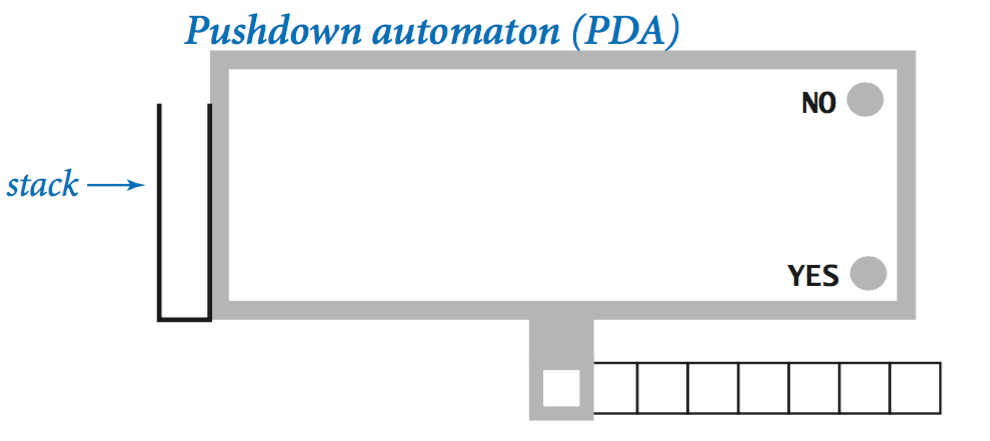It is not difficult to develop a PDA that can recognize binary strings with an equal number of a and b symbols. In the next section, we will consider Turing machines, which is the abstract machine that lies at the heart of computer science. #### Exercises 1. Give an RE that specifies each of the following languages over the binary alphabet. 1. All strings except empty string 2. Contains at least three consecutive bs 3. Starts with a and has odd length, or starts with b and has even length 4. No consecutive bs 5. Any string except bb or bbb 6. Starts and ends with the same symbol 7. Contains at least two as and at most one b Solutions: (a|b)(a|b)*, (a|b)*bbb(a|b)*, a((a|b)(a|b))* | b(a|b)((a|b)(a|b))*, ... #### Creative Exercises 1. Harvester. Write a Pattern and Matcher client Harvester.java that takes a file name (or URL) andd an RE as command-line inputs and prints all substrings in a file that match the RE. 2. Web crawling. Develop a program WebCrawler.java that prints all web pages that can be accessed from the web page given as a command-line argument. 3. Search and replace. Write a filter SearchAndReplace.java that takes an RE and a string str as command-line arguments, reads a string from standard input, replaces all substrings on standard input that match the RE with str, and sends the results to standard output. First solve the problem using the replaceAll() method in Java’s String library; then solve it without using that method. #### Web Exercises (Regular Expressions) 1. Give an RE that specifies each of the following languages over {0, 1}. 1. a or bb or bab 2. only as 3. all binary strings 4. begins with a, ends with a 5. ends with aa Answers: a | bb | bab, a*, (a|b)*, a(a|b)*a | a, (a|b)*aa 2. Write a regular expression to describe inputs over the alphabet {a, b, c} that are in sorted order. Answer: a*b*c*. 3. Write a regular expression for each of the following sets of binary strings. Use only the basic operations. 1. contains at least three consecutive 1s 2. contains the substring 110 3. contains the substring 1101100 4. doesn't contain the substring 110 Answers: (0|1)*111(0|1)*, (0|1)*110(0|1)*, (0|1)*1101100(0|1)*, (0|10)*1*. The last one is by far the trickiest. 4. Write a regular expression for binary strings with at least two 0s but not consecutive 0s. 5. Write a regular expression for each of the following sets of binary strings. Use only the basic operations. 1. has at least 3 characters, and the third character is 0 2. number of 0s is a multiple of 3 3. odd length 4. length is at least 1 and at most 3 Answers: (0|1)(0|1)0(0|1)*, 1* | (1*01*01*01*)*, (0|1)((0|1)(0|1))*, (0|1) | (0|1)(0|1) | (0|1)(0|1)(0|1). 6. For each of the following, indicate how many bit strings of length exactly 1000 are matched by the regular expression: 0(0 | 1)*1, 0*101*, (1 | 01)*. 7. Write a regular expression that matches all strings over the alphabet {a, b, c} that contain: 1. starts and ends with a 2. at most one a 3. at least two a's 4. an even number of a's 5. number of a's plus number of b's is even 8. Find long words whose letters are in alphabetical order, e.g., almost and beefily. Answer: use the regular expression '^a*b*c*d*e*f*g*h*i*j*k*l*m*n*o*p*q*r*s*t*u*v*w*x*y*z*$'.
9. Write a Java regular expression to match phone numbers, with or without area codes. The area codes should be of the form (609) 555-1234 or 555-1234.
10. Find all English words that end with nym.
11. Final all English words that contain the trigraph bze. Answer: subzero.
12. Find all English words that start with g, contain the trigraph pev and end with e. Answer: grapevine.
13. Find all English words that contain the trigraph spb and have at least two r's.
14. Find the longest English word that can be written with the top row of a standard keyboard. Answer: proprietorier.
15. Find all words that contain the four letters a, s, d, and f, not necessarily in that order. Solution: cat words.txt | grep a | grep s | grep d | grep f.
16. Given a string of A, C, T, and G, and X, find a string where X matches any single character, e.g., CATGG is contained in ACTGGGXXAXGGTTT.
17. Write a Java regular expression, for use with Validate.java, that validates Social Security numbers of the form 123-45-6789. Hint: use \d to represent any digit. Answer: [0-9]{3}-[0-9]{2}-[0-9]{4}.
18. Modify the previous exercise to make the - optional, so that 123456789 is considered a legal input.
19. Write a Java regular expression to match all strings that contain exactly five vowels and the vowels are in alphabetical order. Answer: [^aeiou]*a[^aeiou]*e[^aeiou]*i[^aeiou]*o[^aeiou]*u[^aeiou]*
20. Write a Java regular expression to match valid Windows XP file names. Such a file name consists of any sequence of characters other than
 / \ : * ? " < > | 

Additionally, it cannot begin with a space or period.

21. Write a Java regular expression to match valid OS X file names. Such a file name consists of any sequence of characters other than a colon. Additionally, it cannot begin with a period.
22. Given a string s that represents the name of an IP address in dotted quad notation, break it up into its constituent pieces, e.g., 255.125.33.222. Make sure that the four fields are numeric.
23. Write a Java regular expression to describe valid IP addresses of the form a.b.c.d where each letter can represent 1, 2, or 3 digits, and the periods are required. Yes: 196.26.155.241.
24. Write a Java regular expression to match license plates that start with 4 digits and end with two uppercase letters.
25. Write a regular expression to extract the coding sequence from a DNA string. It starts with the ATG codon and ends with a stop codon (TAA, TAG, or TGA). reference
26. Write a regular expression to check for the sequence rGATCy: that is, does it start with A or G, then GATC, and then T or C.
27. Write a regular expression to check whether a sequence contains two or more repeats of the the GATA tetranucleotide.
28. Modify Validate.java to make the searches case insensitive. Hint: use the (?i) embedded flag.
29. Write a Java regular expression to match various spellings of Libyan dictator Moammar Gadhafi's last name using the folling template: (i) starts with K, G, Q, (ii) optionally followed by H, (iii) followed by AD, (iv) optionally followed by D, (v) optionally followed by H, (vi) optionally followed by AF, (vii) optionally followed by F, (vii) ends with I.
30. Write a Java program that reads in an expression like (K|G|Q)[H]AD[D][H]AF[F]I and prints out all matching strings. Here the notation [x] means 0 or 1 copy of the letter x.
31. Why doesn't s.replaceAll("A", "B"); replace all occurrences of the letter A with B in the string s?

Answer: Use s = s.replaceAll("A", "B"); instead The method replaceAll returns the resulting string, but does not change s itself. Strings are immutable.

32. Write a program Clean.java that reads in text from standard input and prints it back out, removing any trailing whitespace on a line and replacing all tabs with 4 spaces.

Hint: use replaceAll() and the regular expression \s for whitespace.

33. Write a regular expression to match all of the text between the text a href =" and the next ". Answer: href=\"(.*?)\". The ? makes the .* reluctant instead of greedy. In Java, use Pattern.compile("href=\\\"(.*?)\\\"", Pattern.CASE_INSENSITIVE) to escape the backslash characters.
34. Write a program Title.java to extract all of the text between the tags <title> and <\title>. The (?i) is makes the match case insensitive. The $2 refers to the second captured subsequence, i.e., the stuff between the title tags.  String pattern = "(?i)()(.+?)()"; String updated = s.replaceAll(pattern, "$2"); 
35. Write a regular expression to match all of the text between <TD ...> and </TD> tags. Answer: <TD[^>]*>([^<]*)</TD>
36. Regular expression for permutations. Find the shortest regular expression (using only the basic operations) you can for the set of all permutations on n elements for n = 5 or 10. For example if n = 3, then the language is abc, acb, bac, bca, cab, cba. Answer: difficult. Solution has length exponential in n.

#### Web Exercises (DFAs and NFAs)

1. Draw a 4-state DFA that accepts the set of all bitstrings ending with 11. (Each state represents whether the input string read in so far ends with 00, 01, 10, or 11.) Draw a 3-state DFA that accomplishes the same task.
2. Draw a DFA for bitstrings with at least one 0 and at least one 1.
3. Draw an NFA that matches all strings that contain either a multiple of three 0s or a multiple of five 1s. Hint: Use 3 + 5 + 1 = 9 states and one epsilon transition.
4. Draw an NFA that recognize the language of all strings that end in aaab.
5. Draw an NFA that recognize the language of all strings whose 4th to the last character is a.
6. Draw an NFA that recognize the language of all strings whose 5th to the last character is a.
7. Give a NFA with 5 accept states. Write an equivalent NFA that has exactly one accept state.
8. Give a NFA with ε-transitions. Write an equivalent NFA that has no ε-transitions.
9. Unary divisibility. Given an DFA that accepts bitstrings with a multiple of three 0s and a multiple of five 1s.
10. DFA for permutations. Find the shortest DFA you can for the set of all permutations on n elements for n = 5 or 10.
11. Mealy and Moore machines. Mealy: DFA with output on each transition edge. Moore: DFA with output on each state.

#### Web Exercises

1. Text-to-speech synthesis. Original motivation for grep. "For example, how do you cope with the digraph ui, which is pronounced many different ways: fruit, guile, guilty, anguish, intuit, beguine?"
2. Boston accent. Write a program to replace all of the r's with h's to translate a sentence like "Park the car in Harvard yard" into the Bostonian version "Pahk the cah in Hahvahd yahd".
3. File extension. Write a program that takes the name of a file as a command line argument and prints out its file type extension. The extension is the sequence of characters following the last .. For example the file sun.gif has the extension gif. Hint: use split("\\."); recall that . is a regular expression meta-character, so you need to escape it.
4. Reverse subdomains. For web log analysis, it is convenient to organize web traffic based on subdomains like wayne.faculty.cs.princeton.edu. Write a program to read in a domain name and print it out in reverse order like edu.princeton.cs.faculty.wayne.
5. Bank robbery. You just witnessed a bank robbery and got a partial license plate of the getaway vehicle. It started with ZD, had a 3 somewhere in the middle and ended with V. Help the police officer write regular expression for this plate.
6. Parsing quoted strings. Read in a text file and print out all quote strings. Use a regular expression like "[^"]*", but need to worry about escaping the quotation marks.
7. Parsing HTML. A >, optionally followed by whitespace, followed by a, followed by whitespace, followed by href, optionally followed by whitespace, followed by =, optionally followed by whitespace, followed by "http://, followed by characters until ", optionally followed by whitespace, then a <.
 < \s* a \s+ href \s* = \s* \\"http://[^\\"]* \\" \s* > 
8. Subsequence. Given a string s, determine whether it is a subsequence of another string t. For example, abc is a subsequence of achfdbaabgabcaabg. Use a regular expression. Now repeat the process without using regular expressions. Answer: (a) a.*b.*c.*, (b) use a greedy algorithm.
9. Huntington's disease diagnostic. The gene that causes Huntington's disease is located on chromosome 4, and has a variable number of repeats of the CAG trinucleotide repeat. Write a program to determine the number of repeats and print will not develop HD If the number of repeats is less than 26, offspring at risk if the number is 37-35, at risk if the number is between 36 and 39, and will develop HD if the number is greater than or equal to 40. This is how Huntington's disease is identified in genetic testing.
10. Repeat finder. Write a program Repeat.java that takes two command line arguments, and finds the maximum number of repeats of the first command line argument in the file specified by the second command line argument.
11. Character filter. Given a string t of bad characters, e.g. t = "!@#$%^&*()-_=+", write a function to read in another string s and return the result of removing all of the bad characters.  String pattern = "[" + t + "]"; String result = s.replaceAll(pattern, "");  12. Wildcard pattern matcher. Without using Java's built in regular expressions, write a program Wildcard.java to find all words in the dictionary matching a given pattern. The special symbol * matches any zero or more characters. So, for example the pattern "w*ard" matches the word "ward" and "wildcard". The special symbol . matches any one character. Your program should read the pattern as a command line parameter and the list of words (separated by whitespace) from standard input. 13. Wildcard pattern matcher. Repeat the previous exercise, but this time use Java's built in regular expressions. Warning: in the context of wildcards, * has a different meaning than with regular expressions. 14. Password validator. Suppose that for security reasons you require all passwords to have at least one of the following characters  ~ ! @ #$ % ^ & * | 
Write a regular expression for use with String.matches that returns true if and only if the password contains one of the required characters. Answer: "^[^~!@#$%^&*|]+$"
15. Alphanumeric filter. Write a program Filter.java to read in text from standard input and eliminate all characters that are not whitespace or alpha-numeric. Answer here's the key line.
 String output = input.replaceAll("[^\\s0-9a-zA-Z]", ""); 
16. Converting tabs to spaces. Write a program to convert all tabs in a Java source file to 4 spaces.
17. Parsing delimited text files. A popular way to store a database is in a text file with one record per line, and each field separated by a special character called the delimiter.
 19072/Narberth/PA/Pennsylvania 08540/Princeton/NJ/New Jersey 
Write a program Tokenizer.java that reads in a delimiter character and the name of a file, and creates an array of tokens in the file.
18. Parsing delimited text files. Repeat the previous exercise, but use the String library method split().
19. PROSITE to Java regular expression. Write a program to read in a PROSITE pattern and print out the corresponding Java regular expression.
20. Misspellings. Write a Java program to verify that this list of common misspellings adapted from Wikipedia contains only lines of the form
 misdemenors (misdemeanors) mispelling (misspelling) tennisplayer (tennis player) 

where the first word is the misspelling and the string in parentheses is a possible replacement.

21. Comment stripper. Write a program CommentStripper.java that reads in a Java (or C++) program from standard input, removes all comments, and prints the result to standard output. This would be useful as part of a Java compiler. It removes /* */ and // style comments using a 5 state finite state automaton. It is meant to illustrate the power of DFAs, but to properly strip Java comments, you would need a few more states to handle extra cases, e.g., quoted string literals like s = "/***//*". The picture below is courtesy of David Eppstein.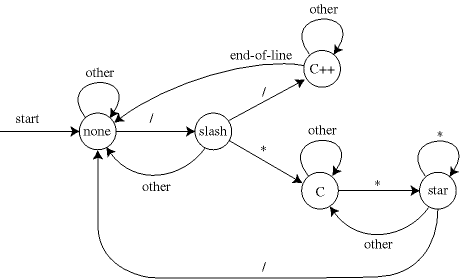22. Improved comment stripper. Modify CommentStripper.java to properly handle characters within quote strings.
23. Sleep on it. An experiment conducted by German neurologists and documented in Nature, volume 427 (2004), p. 352 hypothesizes that students who get more sleep are able to solve tricky problems better than students who are sleep deprived. The problem they used involves a string consisting of the three digits 1, 4, and 9. "Comparing" two digits that are the same yields the original digit; comparing two digits that are different yields the missing digit. For example f(1, 1) = 1, f(4, 4) = 4, f(1, 4) = 9, f(9, 1) = 4. Compare the first two digits of the input string, and then repeatedly compare the current result with the next digit in the string. Given a specific string, what number do you end up with? For example, if the input is string 11449494, you end up with 9.
 1 1 4 4 9 4 9 4 1 9 1 4 4 1 9